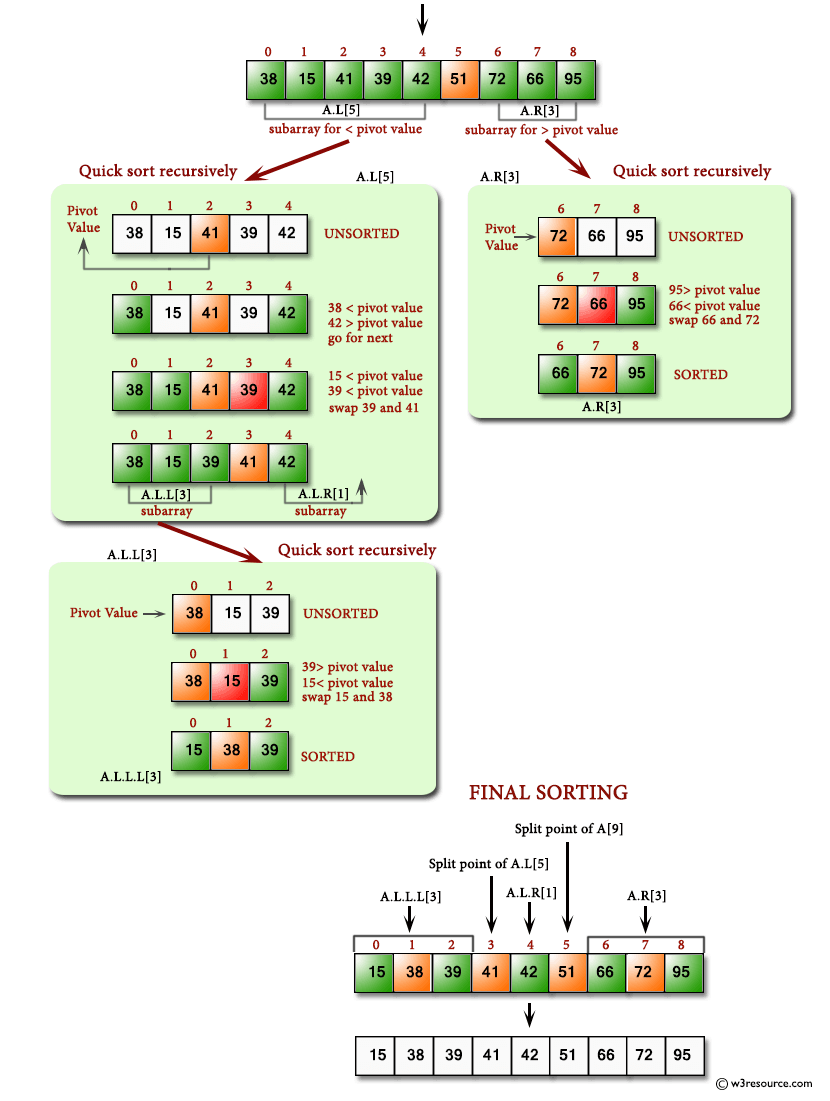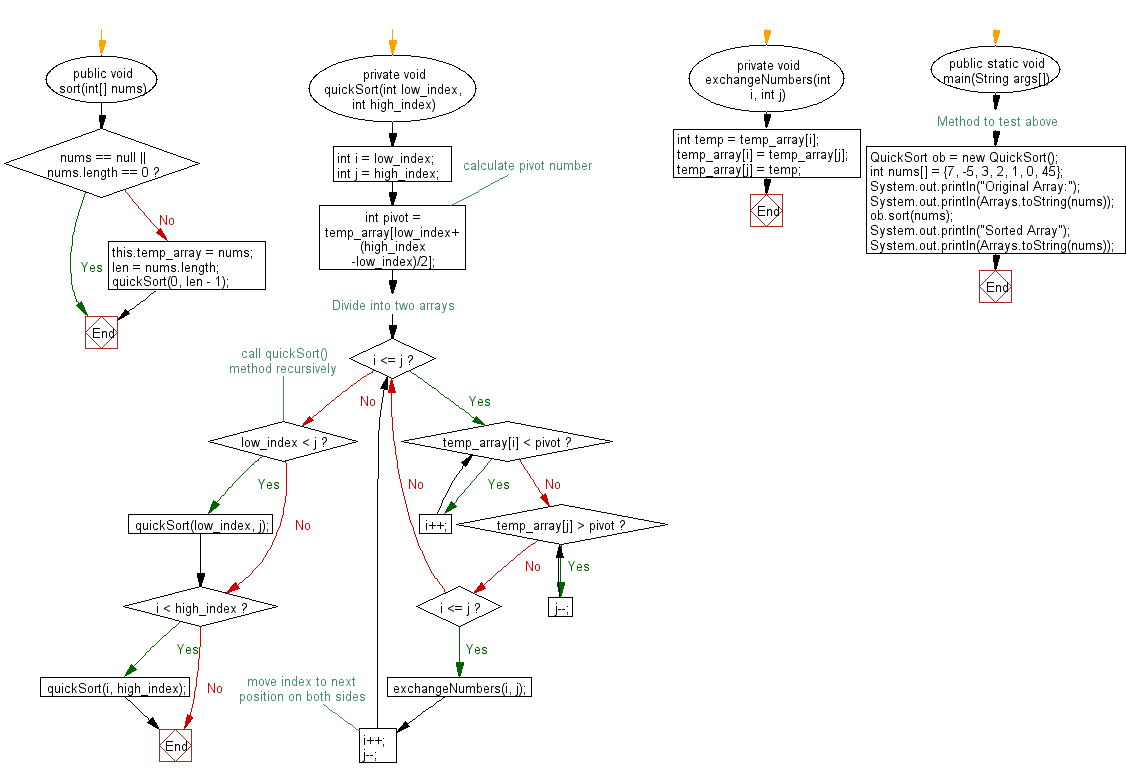﻿ Java exercises: Quick sort Algorithm - w3resource# Java Exercises: Quick sort Algorithm

## Java Sorting Algorithm: Exercise-1 with Solution

Write a Java program to sort an array of given integers using Quick sort Algorithm.

Quick sort is a comparison sort, meaning that it can sort items of any type for which a "less-than" relation (formally, a total order) is defined.

Pictorial presentation - Quick Sort algorithm :Sample Solution:

Java Code:

``````import java.util.Arrays;
public class QuickSort {

private int temp_array[];
private int len;

public void sort(int[] nums) {

if (nums == null || nums.length == 0) {
return;
}
this.temp_array = nums;
len = nums.length;
quickSort(0, len - 1);
}
private void quickSort(int low_index, int high_index) {

int i = low_index;
int j = high_index;
// calculate pivot number
int pivot = temp_array[low_index+(high_index-low_index)/2];
// Divide into two arrays
while (i <= j) {
while (temp_array[i] < pivot) {
i++;
}
while (temp_array[j] > pivot) {
j--;
}
if (i <= j) {
exchangeNumbers(i, j);
//move index to next position on both sides
i++;
j--;
}
}
// call quickSort() method recursively
if (low_index < j)
quickSort(low_index, j);
if (i < high_index)
quickSort(i, high_index);
}

private void exchangeNumbers(int i, int j) {
int temp = temp_array[i];
temp_array[i] = temp_array[j];
temp_array[j] = temp;
}

// Method to test above
public static void main(String args[])
{
QuickSort ob = new QuickSort();
int nums[] = {7, -5, 3, 2, 1, 0, 45};
System.out.println("Original Array:");
System.out.println(Arrays.toString(nums));
ob.sort(nums);
System.out.println("Sorted Array");
System.out.println(Arrays.toString(nums));
}
}
```
```

Sample Output:

```Original Array:
[7, -5, 3, 2, 1, 0, 45]
Sorted Array
[-5, 0, 1, 2, 3, 7, 45]
```

Flowchart:Java Code Editor:

What is the difficulty level of this exercise?

﻿

## Java: Tips of the Day

Array vs ArrayLists:

The main difference between these two is that an Array is of fixed size so once you have created an Array you cannot change it but the ArrayList is not of fixed size. You can create instances of ArrayLists without specifying its size. So if you create such instances of an ArrayList without specifying its size Java will create an instance of an ArrayList of default size.

Once an ArrayList is full it re-sizes itself. In fact, an ArrayList is internally supported by an array. So when an ArrayList is resized it will slow down its performance a bit as the contents of the old Array must be copied to a new Array.

At the same time, it's compulsory to specify the size of an Array directly or indirectly while creating it. And also Arrays can store both primitives and objects while ArrayLists only can store objects.

Ref: https://bit.ly/3o8L2KH